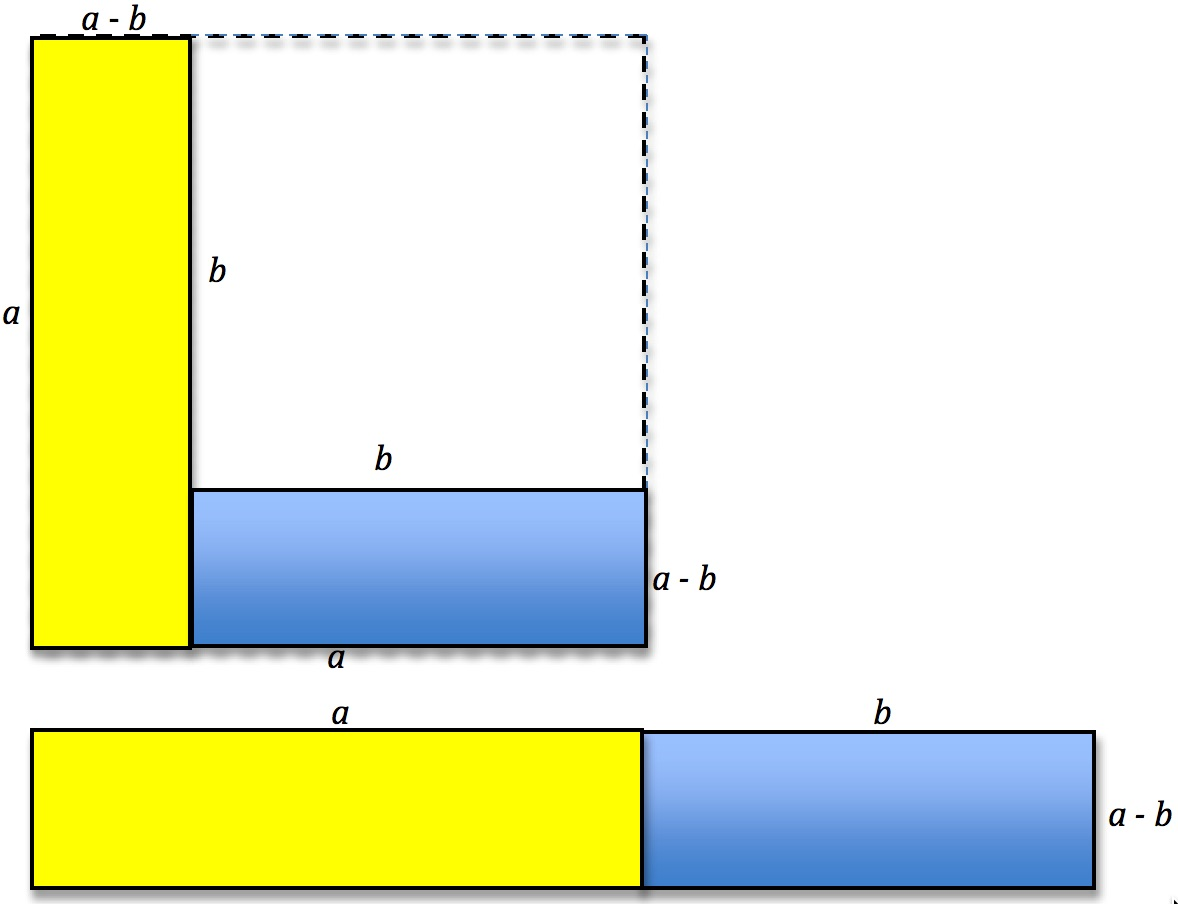What do you see in this image? What is the area of the bigger square edged by dotted lines? What is left if you take away a square with sides of length b? What can you deduce from this?

How many numbers between 1 and 30 can you express as the difference of two squares? For example $$7=4^2-3^2, 20=6^2-4^2$$ and $$25=5^2-0^2$$.

What do you notice about the difference between squares of consecutive numbers?

What about the difference between the squares of numbers which differ by 2? By 3? By 4…?
When is the difference between two square numbers odd?
And when is it even?

What do you notice about the numbers you CANNOT express as the difference of two perfect squares?

Can you prove any of your findings?

Click here for Notes for Teachers. Thank you to NRICH for some of the ideas here. For more challenges about the differences of two squares see Plus Minus on the NRICH website.